### 8.5.1.1 An approximate cover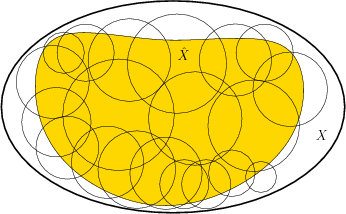Figure 8.15 illustrates the notion of an approximate cover, which will be used to represent the funnel domains. Let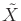denote a subset of a state space. A cover ofis a collection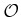of sets for which

1.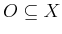for each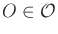.
2.is a subset of the union of all sets in the cover: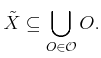(8.50)

Let eachbe called a neighborhood. The notion of a cover was actually used in Section 8.3.2 to define a smooth manifold using a cover of coordinate neighborhoods.

In general, a cover allows the following:

1. Any number of neighborhoods may overlap (have nonempty intersection).
2. Any neighborhood may contain points that lie outside of.
A cell decomposition, which was introduced in Section 6.3.1, is a special kind of cover for which the neighborhoods form a partition of, and they must fit together nicely (recall Figure 6.15).

So far, no constraints have been placed on the neighborhoods. They should be chosen in practice to greatly simplify the design of a navigation function over each one. For the original motion planning problem, cell decompositions were designed to make the determination of a collision-free path trivial in each cell. The same idea applies here, except that we now want to construct a feedback plan. Therefore, it is usually assumed that the cells have a simple shape.

A cover is called approximate ifis a strict subset of. Ideally, we would like to develop an exact cover, which implies that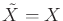and each neighborhood has some nice property, such as being convex. Developing such covers is possible in practice for state spaces that are either low-dimensional or exhibit some special structure. This was observed for the cell decomposition methods of Chapter 6.

Consider constructing an approximate cover for. The goal should be to cover as much ofas possible. This means that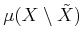should be made as small as possible, in which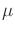denotes Lebesgue measure, as defined in Section 5.1.3. It is also desirable to ensure thatpreserves the connectivity of. In other words, if a path between two points exists in, then it should also exist in.

Steven M LaValle 2020-08-14